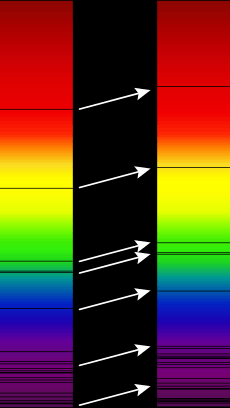# Hubble's law facts for kids

Kids Encyclopedia FactsAbsorption lines in the visible spectrum of a supercluster of distant galaxies (right), as compared to absorption lines in the optical spectrum of the Sun (left). Arrows indicate redshift. Wavelength increases up towards the red and beyond (frequency decreases).

Hubble's law or Hubble—Lemaître's law is the name for the observation that:

1. All objects observed in deep space have a doppler shift-measured velocity relative to Earth, and to each other;
2. The doppler-shift-measured velocity of galaxies moving away from Earth, is proportional to their distance from the Earth and all other interstellar bodies.

In effect, the space-time volume of the observable universe is expanding and Hubble's law is the direct physical observation of this. It is the basis for believing in the expansion of the universe and is evidence often cited in support of the Big Bang model.

Although widely attributed to Edwin Hubble, the law was first derived from the General Relativity equations by Georges Lemaître in a 1927 article. There he proposed that the Universe is expanding, and suggested a value for the rate of expansion, now called the Hubble constant. Two years later Edwin Hubble confirmed the existence of that law and determined a more accurate value for the constant that now bears his name. The recession velocity of the objects was inferred from their redshifts, many measured earlier by Vesto Slipher in 1917 and related to velocity by him.

The law is often expressed by the equation v = H0D, with H0 the constant of proportionality (the Hubble constant) between the "proper distance" D to a galaxy and its velocity v (see Uses of the proper distance). H0 is usually quoted in (km/s)/Mpc, which gives the speed in km/s of a galaxy 1 megaparsec (3.09×1019 km) away. The reciprocal of H0 is the Hubble time.

A recent 2011 estimate of the Hubble constant, which used a new infrared camera on the Hubble Space Telescope (HST) to measure the distance and redshift for a collection of astronomical objects, gives a value of H0 = 73.8 ± 2.4 (km/s)/Mpc. An alternate approach using data from galactic clusters gave a value of H0 = 67.0 ± 3.2 (km/s)/Mpc.

A number of other methods have been used, giving figures between 70 and 72 (km/s)/Mpc. A recent (2016) method using the oldest light in the universe suggests the Hubble Constant value was 66.53km/s per megaparsec soon after the expansion began. This implies that the rate of expansion has been increasing.

## Images for kidsHubble's law Facts for Kids. Kiddle Encyclopedia.